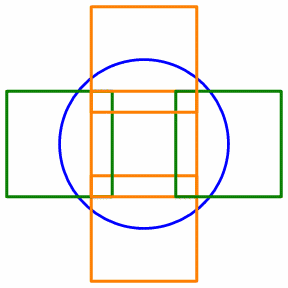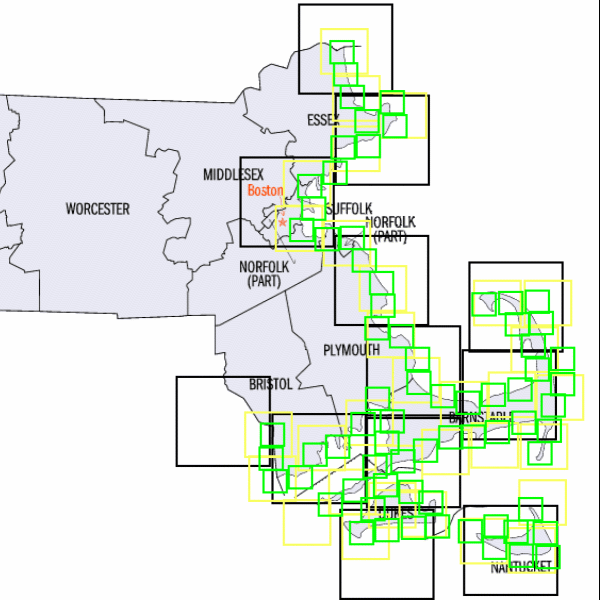Fall 2003

# Mathematics Math21b Fall 2003

## Linear Algebra and Differential Equations

Office: SciCtr 434
Email: knill@math.harvard.edu# The Dimension of the Massachusetts Coastline

(An example of a data fitting problem)The number of squares of length 1/n needed to cover the circle grows linearly with n. The circle has dimension 1. The number of squares of length 1/n needed to cover the dis grows like n2. The disk has dimension 2.

 On the other hand, the number of squares of length 1/n needed to cover the coast of Massachusetts grows like nd with a number d between 1 and 2. The curve appears to be a fractal. The measurement of the number of squares is a counting exercice. The computation of the growth rate needs a linear fit. ( More Details (PDF))PDF File with information
Back to the main page